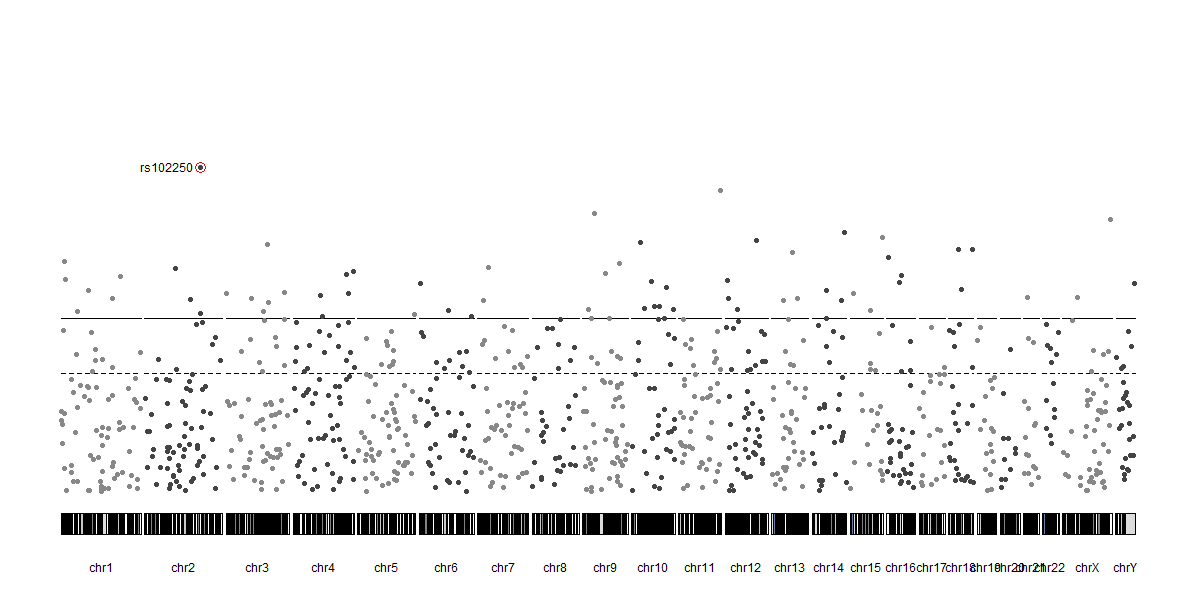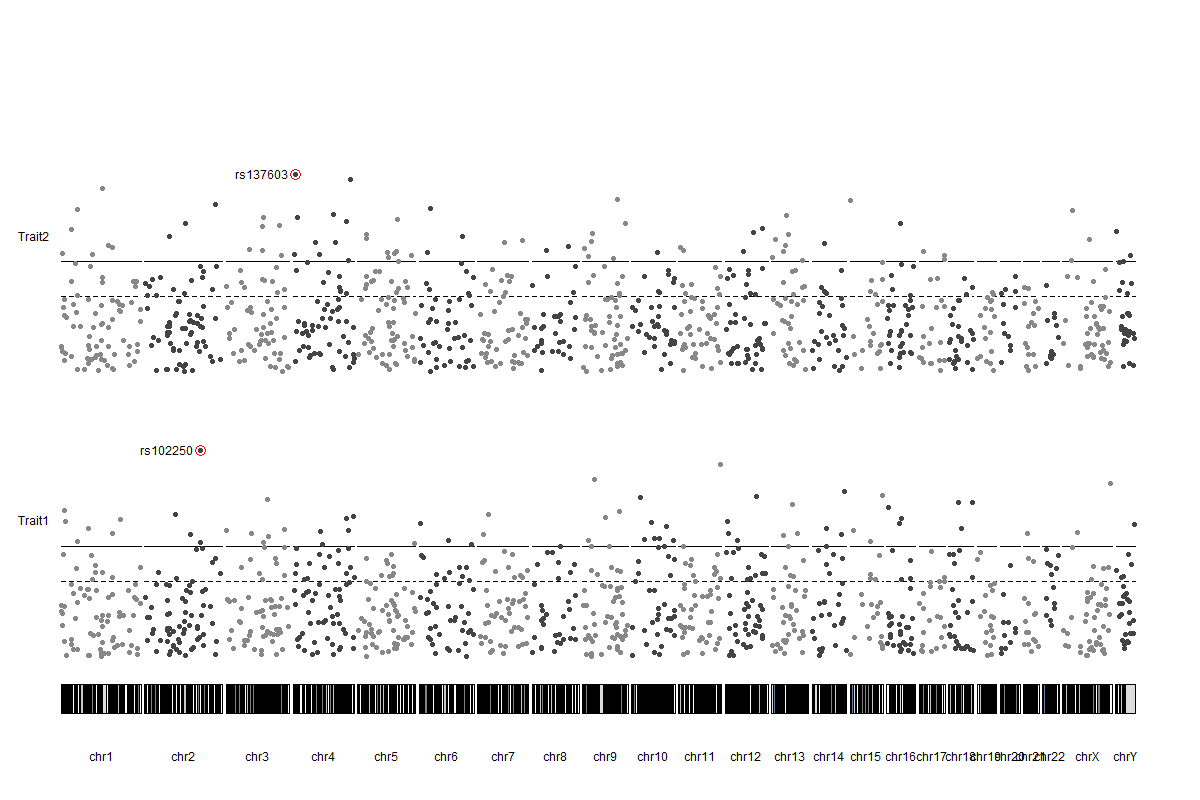Labelling SNPs in a stacked manhattan plot with karyoploteR
1
1
Entering edit mode
23 months ago
jsreddy ▴ 20

When combining multiple GWAS results into a stacked manhattan plot using KaryoploteR, how do you label top SNPs in each set of GWAS results? Instructions provided for labelling a single manhattan plot did not work for the stacked manhattan.

manhattan gwas plot karyoploteR • 1.9k views
0
Entering edit mode
23 months ago
bernatgel ★ 3.4k

Hi @jsreddy

You should be able to apply the snp labelling code to the stacked manhattan using almost the same code provided in the example. The key is to give the same autotrack you use for kpPlotManhattan to the functions used for labeling (kpPoints and kpText, for example).

Does it make sense? You should replicate the labeling code as you replicate the call to kpPlotManhattan and give all functions plotting in the same "track" the same autotrack configuration.

If this is not clear yet, I can provide a small example. Just ask.

Hope this helps

Bernat

0
Entering edit mode

Thank you @bernatgel for your response, your explanation was absolutely clear. I used the autotrack with kpText() to make sure labels are linked to the corresponding set of results. But I ended up generating a plot with all the labels at the top of the plot.

Code snippet/example can be extremely helpful. I can also send you my code/figure if that helps.

Thanks,
Joseph.

2
Entering edit mode

Ok, here's the code then. I've created a small example with two stacked manhattan plots for two traits (all simulated data) with a label on the top snp for each one. All code and images can be found also on github.

### Create the simulated data

Create the simulated data with regioneR::createRandomRegions (available if you load karyoploteR) and a couple of random number generations. Select the two top snps and compute their y position.

library(karyoploteR)

set.seed(1234)
snps <- createRandomRegions(nregions = 1000, length.mean = 1, length.sd = 0,
snps$rs <- paste0("rs", round(runif(1000, min = 1000, max = 200000))) #Trait 1 snps$pval1 <- 10^(-1*abs(rnorm(n = 1000, 1,sd = 4 )))
top1 <- which.min(snps$pval1) top1.y <- -1 * log10(snps[top1]$pval1)
#Trait 2
snps$pval2 <- 10^(-1*abs(rnorm(n = 1000, 1,sd = 4 ))) top2 <- which.min(snps$pval2)
top2.y <- -1 * log10(snps[top2]$pval2)  ### One Manhattan plot Plot only Trait 1 and label the top snp. kp <- plotKaryotype(genome="hg19", plot.type=4) kpPlotManhattan(kp, data = snps, pval = snps$pval1, ymin=0, ymax=18)
kpPoints(kp, data=snps[top1], y = top1.y, pch=1, cex=2, col="red", ymin=0, ymax=18)
kpText(kp, data = snps[top1], y=top1.y, labels = snps[top1]$rs, pos=2, ymin=0, ymax=18)  And this is the result## Two stacked Manhattan plots To stack the two manhattan plots we simply add r0=autotrack(1,2) to the code plotting the first trait and r0=autotrack(2,2)to the code plotting the second track. I've also added a couple of labels for clarity. kp <- plotKaryotype(genome="hg19", plot.type=4) kpAddLabels(kp, labels = "Trait1", r0=autotrack(1,2)) kpPlotManhattan(kp, data = snps, pval = snps$pval1, ymin=0, ymax=18, r0=autotrack(1,2))
kpPoints(kp, data=snps[top1], y = top1.y, pch=1, cex=2, col="red", ymin=0, ymax=18, r0=autotrack(1,2))
kpText(kp, data = snps[top1], y=top1.y, labels = snps[top1]$rs, pos=2, ymin=0, ymax=18, r0=autotrack(1,2)) kpAddLabels(kp, labels = "Trait2", r0=autotrack(2,2)) kpPlotManhattan(kp, data = snps, pval = snps$pval2, ymin=0, ymax=18, r0=autotrack(2,2))
kpPoints(kp, data=snps[top2], y = top2.y, pch=1, cex=2, col="red", ymin=0, ymax=18, r0=autotrack(2,2))
kpText(kp, data = snps[top2], y=top2.y, labels = snps[top2]\$rs, pos=2, ymin=0, ymax=18, r0=autotrack(2,2))


And the image with the two stacked Manhattan plots is thisHope this helps :)

Bernat

0
Entering edit mode

Thank you, Bernat! Will give this a try. Appreciate your response!

Joseph.

1
Entering edit mode

Bernat,

Code worked like a charm. Thank you! I learned that you have to include ylim parameters every step of the way. I wasn't doing that before and it was going haywire.

I have a follow-up question, is there a way to just do kpPoints and kpText of the top SNP that reaches a certain p-value threshold in each chromosome? With your current code, if I have multiple SNPs in a CHR that meet the threshold, all those SNPs are being plotted and the text becomes hard to read since it overlaps. I only want to show the top hit per CHR. Is there a flag for this?

Thanks,
Joseph.

0
Entering edit mode

Sure, you can do this, but not with a flag.

The key here is the filtering to select the snps you want in top1 or top2. In the code above there's a selection for the top SNP in the dataset. You should first select the top SNP in each chromosome (e.g. split the SNPs by chromosome, select the top one in each chromosome and finally discard any SNP below a certain value). And then use the code above to plot the labels.

I'm developing a new package specifically designed for manhattan plots that will make annotation muuuch more simple, but it's not ready yet.

If you need more help with this, just ask.

Bernat